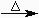Chemical Reactions - Experiment

## The Experiment

Below are ten potential reactions. For each, read how to perform the reaction and then examine the results. Record all of your observations (your observations should include descriptions of both the reactants and products). Next answer the questions based on your observations and the information provided in the background. If told to do so by your instructor you should read about writing ionic equations and then write net ionic equations for only reactions A, B, D, E, G and H.

Clicking on "see results" will show the reactants and the products of the reaction. If you would like to see a bigger picture of a reactant, click on its name in the procedure description. For a bigger picture of the products, click on the word "products" at the end of the procedure description. Note that the same picture is used to represent all of the colorless solutions.

 A. zinc + hydrochloric acidsee results Drop a small piece of zinc into a test tube containing 1-2 ml of 1.0 M hydrochloric acid solution. (products) 1. Complete and balance the equation. 2. Classify the reaction. 3. What gas was formed? B. iron + copper (II) sulfatesee results Drop a small piece of iron into a test tube containing 1-2 ml of 1.0 M copper (II) sulfate solution. (products) 1. Complete and balance the equation. 2. Classify the reaction. 3. The blue color in the copper sulfate solution is due to the presence of what ion? 4. Predict the color of a copper nitrate solution. C. ammonia gas + hydrogen chloride gassee results Put 1-2 ml of concentrated ammonia solution into one test tube and 1-2 ml of concentrated hydrochloric acid solution into a second. Bring the mouths of the tubes near each other. (products) 1. Complete and balance the equation. 2. Classify the reaction. 3. What is the difference between hydrochloric acid and hydrogen chloride? 4. The product of this reaction is a solid. Why does it look like smoke? D. sodium carbonate + calcium chloridesee results Add 1-2 ml of a 0.1 M sodium carbonate solution to a test tube containing 1-2 ml of a 0.1 M calcium chloride solution. (products) 1. Complete and balance the equation. 2. Classify the reaction. 3. The solid formed in this reaction is what compound? E. copper + hydrochloric acidsee results Drop a small piece of copper into a test tube containing 1-2 ml of 1.0 M hydrochloric acid solution. (products) 1. Complete and balance the equation. 2. Classify the reaction. F. copper sulfate pentahydratesee results Put a small amount of copper sulfate pentahydrate in a crucible and heat gently with a Bunsen burner. When the reaction is complete, allow the crucible to cool (products) and then add a drop of water to the product. (products) 1. Complete and balance the equation. 2. Classify the reaction. 3. Write the equation for the reaction that occurs when a drop of water is added to products. G. potassium chloride + sodium carbonatesee results Add 1-2 ml of a 0.1 M potassium chloride solution to a test tube containing 1-2 ml of a 0.1 M sodium carbonate solution. (products) 1. Complete and balance the equation. 2. Classify the reaction. H. lead (II) nitrate + potassium iodidesee results Add 1-2 ml of a 0.1 M lead (II) nitrate solution to a test tube containing 1-2 ml of a 0.1 M potassium iodide solution. (products) 1. Complete and balance the equation. 2. Classify the reaction. 3. What is the identity of the solid formed in this reaction? I. magnesium metal + oxygensee results Put a small amount of magnesium metal in a crucible and heat gently with a Bunsen burner. Be careful when heating as burning magnesium produces a blinding light. If the magnesium ignites, the lid is quickly placed on the crucible to extinguish the flame. (products) 1. Complete and balance the equation. 2. Classify the reaction. J. potassium hydrogen carbonate + hydrochloric acidsee results Add 1-2 ml of a 0.1 M hydrochloric acid solution to a test tube containing a small amount of potassium hydrogen carbonate. (products) 1. Complete and balance the equation. 2. Classify the reaction. 3. What gas is formed? K. ammonium carbonatesee results Place a small amount of ammonium carbonate in a test tube. Attach a piece of rubber tubing to the test tube and gently heat the tube. Bubble the gases produced through a saturated calcium hydroxide solution and then through a phenolphthalein indicator solution. The solid formed when the products of the reaction are passed through the calcium hydroxide solution is calcium carbonate. Phenolphthalein is an acid-base indicator that is bright pink or purple in a basic solution. 1. Complete and balance the reaction. 2. Classify the reaction.
Continue on to get some additional practice.